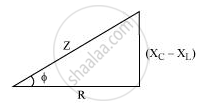Advertisement Remove all ads

# A Series Lcr Circuit is Connected to a Source Having Voltage V = Vm Sin ωT. Derive the Expression for the Instantaneous Current I and Its Phase Relationship to the Applied Voltage. - Physics

Advertisement Remove all ads
Advertisement Remove all ads
Advertisement Remove all ads

A series LCR circuit is connected to a source having voltage v = vm sin ωt. Derive the expression for the instantaneous current I and its phase relationship to the applied voltage.

Obtain the condition for resonance to occur. Define ‘power factor’. State the conditions under which it is (i) maximum and (ii) minimum.

Advertisement Remove all ads

#### Solution

v = vm sin ωt

Let the current in the circuit be led the applied voltage by an angleΦ.

i= i_m sin(omegat +phi)

The Kirchhoff’s voltage law givesL ((di)/dt +Ri +q/C = v).

It is given that v = vm sin ωt (applied voltage)

L(d^2q)/(dt^2) +R(dq)/(dt) +q/C = v_m sinomegat    ...... (1)

On solving the equation, we obtain

q = q_m sin(omegat + theta)

(dp)/(dt) = q_momega cos(omegat +theta)

((d^2)q)/(dt^2)  = -q_momega^2 sin(omegat +theta)

On substituting these values in equation (1), we obtain

q_momega[R cos(omegat +theta)+ (X_c -X_L)sin(omegat +theta)] = v_msinomegat

X_c = 1/(omegaC)  X_c = omegaL

Z = sqrt(R^2 +(X_c - X_L)^2

q_momegaZ[R/Z cos(omegat+theta)+((X_c -X_L))/Z sin (omegat+theta)] = v_m sin omegat         ........... (2)

Let cos phi = R/2 and (X_c -X_L)/Z = sinphi

This gives

tan phi = (X_c - X_L)/R

On substituting this in equation (2), we obtain

q_momegaZcos (omegat +theta -phi) = v_msinomegat

On comparing the two sides, we obtain

V_m = q_momegaZ = i+mZ

i_m = q_momega

and (theta-phi) = -pi/2

I = (dp)/(dt ) =q_momega cos (omegat+theta)

=i_m cos(omegat+theta)

Or

i = i_m sin(omegat +theta)

Where,i_m = (v_m)/Z = (v_m)/(sqrt(R^2 +(X_c - X_L)^2)

And

phi = tan^-1((X_c -X_L)/R)

The condition for resonance to occur

i_m = v_m/sqrt(R^2 +(X_C - X_L)^2)

For resonance to occur, the value of im has to be the maximum.

The value of im will be the maximum when

X_c = X_L

1/(omega C) = omegaL

omega^2 = 1/(LC)

omega = 1/(sqrtLC)

2pif = 1/sqrt(LC)

f = 1/(02pisqrt(LC)

Power factor = cos ΦWhere,cosphi = R/Z = R/(sqrt(R^2 +(X_c- X_L)^2)

(i) Conditions for maximum power factor (i.e., cos Φ = 1)

• XC = XL

Or

• R = 0

(ii) Conditions for minimum power factor

• When the circuit is purely inductive

• When the circuit is purely capacitive

Concept: Ac Voltage Applied to a Series Lcr Circuit
Is there an error in this question or solution?

#### Video TutorialsVIEW ALL 

Advertisement Remove all ads
Share
Notifications

View all notifications

Forgot password?